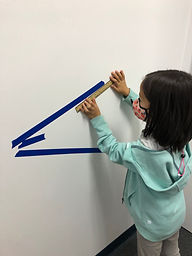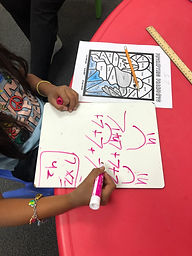## Ms. Hiral

### Target 1​

###### Lesson Type:

New

Measurment

:

Comparison

Measure and compare objects in standard units.

###### 1:

Understand how to measure length using a standard unit (centimeter, inches).

###### 2:

Identify that time can be measured with calendars, clocks, stopwatches, hourglasses, etc.

###### 3:

Identify that weight can be measured with scales, balances, etc.

###### 4:

Express, with a unit number, the length difference of objects in terms which is longer or shorter.

2nd

###### Vocabulary:

Length, Centimeter, Inch, Weight, Time, Measure

Activities:

Students measured different lengths of objects in inches and centimeters.### Home Exploration

###### Guiding Questions:## Absent Students:

### Target 2

:

###### 1:

Understand that multiplication can be represented with arrays, repeated addition, and skip counting.

###### 2:

Understand that the same process is utilized to multiply 3 or more whole numbers that is used to multiply only two numbers (i.e., 2x3x4 = 2 groups of 3 in 6, and 4 groups of 6 is 24).

###### 3:

Interpret products of whole numbers as the total number of objects arranged into equal groupings.

2nd

###### Vocabulary:

Activities:

1. Students played a game called Multiplication War.

2. Students worked on the "Petrifying Product" worksheet. Students solved all the multiplication equations and colored in the spaces according to the key given.### Home Exploration

###### Guiding Questions:### Target 3

:

###### Vocabulary:

Activities:### Home Exploration